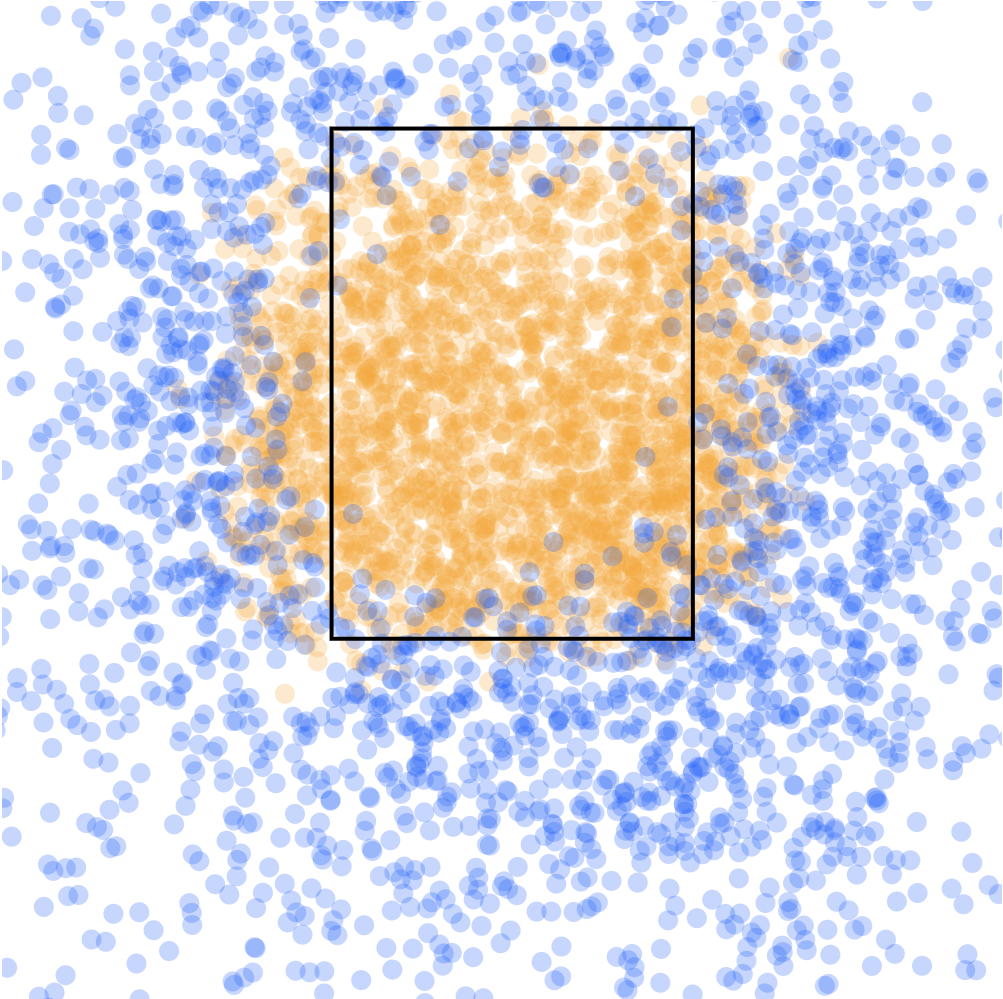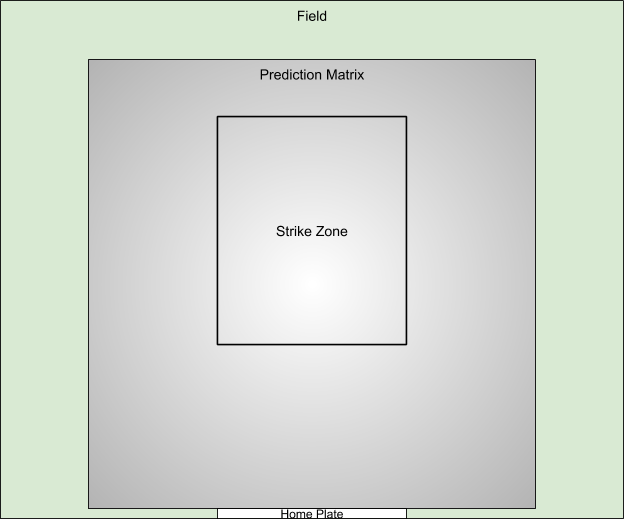TensorFlow.js ·Predicting balls and strikes using TensorFlow.js
July 25, 2018
Posted by Nick Kreeger

In this post we’ll be using TensorFlow.js, D3.js, and the power of the web to visualize the process of training a model to predict balls (blue areas) and strikes (orange areas) from baseball data. As we go, we’ll visualize the strike zone the model understands throughout training. You can run this model entirely in the browser by visiting this Observable notebook.

If you’re not familiar with the strike zone in baseball here’s an article with details.The GIF above visualizes the neural network learning to call balls (blue areas) and strikes (orange areas). After each training step, the heatmap updates with the predictions from the model.
Run this model directly in your browser using Observable.

Today’s professional sports environment is packed with large amounts of data. This data is being applied to all sorts of use cases by teams, hobbyists, and fans. Thanks to frameworks like TensorFlow — these datasets are ready for application of machine learning.

#### MLBAM’s PITCHf/x

Major League Baseball Advanced Media (MLBAM) publishes a large dataset available for research to the public. This dataset contains sensor information about pitches thrown in MLB games for the last several years. Using this dataset, I’ve curated a training set containing 5,000 samples (2,500 balls and 2,500 strikes).

Here is a sample of the first few fields from the training data:

Here is what the training data looks like when plotted against the strike zone. Blue dots are labeled balls and orange dots are labeled as strikes (as called by a major league umpire):### Building the model with TensorFlow.js

TensorFlow.js brings machine learning to JavaScript and the web. We’re going to use this awesome framework to build a deep-neural network model. This model will be able to call balls and strikes with the precision of a major league umpire.

#### Input

This model is trained on the following fields from PITCHf/x:
• Coordinates where the ball crossed home plate (‘px’ and ‘pz’).
• Which side of the plate the batter was standing on.
• The height of the strike zone (the batter’s torso) in feet.
• The height of the bottom of the strike zone (the batter’s knees) in feet.
• The actual label from the pitch (ball or strike) as called by an umpire.

#### Architecture

This model will be defined by using the TensorFlow.js Layers API. The Layers API is based on Keras and will be familiar to those who have used that framework before:
``````const model = tf.sequential();

// Two fully connected layers with dropout between each:
model.add(tf.layers.dense({units: 24, activation: 'relu', inputShape: }));

// Only two classes: "strike" and "ball":

model.compile({
loss: 'categoricalCrossentropy',
metrics: ['accuracy']
});``````

The curated training set is available over a GitHub gist. This dataset needs to be downloaded in order to begin converting the CSV data into a format TensorFlow.js uses for training.
``````const data = [];
csvData.forEach((values) => {
// 'logit' data uses the 5 fields:
const x = [];
x.push(parseFloat(values.px));
x.push(parseFloat(values.pz));
x.push(parseFloat(values.sz_top));
x.push(parseFloat(values.sz_bot));
x.push(parseFloat(values.left_handed_batter));
// The label is simply 'is strike' or 'is ball':
const y = parseInt(values.is_strike, 10);
data.push({x: x, y: y});
});
// Shuffle the contents to ensure the model does not always train on the same
// sequence of pitch data:
tf.util.shuffle(data);``````
After parsing the CSV data, the JS types will need to be converted into Tensor batches for training and evaluation. See the code lab for more details on this process. The TensorFlow.js team is working on a new Data API to make this ingestion much easier in the future.

### Training the model

Let’s put this all together. The model is defined, training data is ready, and now we’re ready to begin training. The following async method trains one batch of training samples and updates the heatmap:
``````// Trains and reports loss+accuracy for one batch of training data:
async function trainBatch(index) {
const history = await model.fit(batches[index].x, batches[index].y, {
epochs: 1,
shuffle: false,
validationData: [batches[index].x, batches[index].y],
batchSize: CONSTANTS.BATCH_SIZE
});

// Don't block the UI frame by using tf.nextFrame()
await tf.nextFrame();
updateHeatmap();
await tf.nextFrame();
}``````

### Visualizing the model’s accuracy

The heatmap is built using a prediction matrix from a sample 4ft x 4ft grid placed evenly above home plate. This matrix is passed into the model after each training step to check how accurate the model is. The results of that prediction are rendered as a heatmap using the D3 library.

#### Building the prediction matrix

The prediction matrix used in the heatmap starts at the middle of home plate and extends 2 feet to the left and right. It also ranges from the bottom of home plate to 4 feet high. The sample strike zone ranges between 1.5 and 3.5 feet above home plate. The visual below helps visualize these 2d panes:This visual shows where the strike zone and prediction matrix relate to home plate and the field of play.

#### Using the prediction matrix with the model

After each a batch is trained in the model, the prediction matrix is passed into the model to ask for ball or strike predictions in the matrix:
``````function predictZone() {
const predictions = model.predictOnBatch(predictionMatrix.data);
const values = predictions.dataSync();

// Sort each value so the higher prediction is the first element in the array:
const results = [];
let index = 0;
for (let i = 0; i < values.length; i++) {
let list = [];
list.push({value: values[index++], strike: 0});
list.push({value: values[index++], strike: 1});
list = list.sort((a, b) => b.value - a.value);
results.push(list);
}
return results;
}``````

#### Heatmap with D3

The prediction results can now be visualized using D3. Each element from the 50x50 grid will be rendered as a 10px x 10px rect in SVG. The color of each rect will depend on the prediction result (ball or strike) and how sure the model was of that result (scale from 50%-100%). The following code snippet shows how data is updated from a D3 svg rect grouping:
``````function updateHeatmap() {
rects.data(generateHeatmapData());
rects
.attr('x', (coord) => { return scaleX(coord.x) * CONSTANTS.HEATMAP_SIZE; })
.attr('y', (coord) => { return scaleY(coord.y) * CONSTANTS.HEATMAP_SIZE; })
.attr('width', CONSTANTS.HEATMAP_SIZE)
.attr('height', CONSTANTS.HEATMAP_SIZE)
.style('fill', (coord) => {
if (coord.strike) {
return strikeColorScale(coord.value);
} else {
return ballColorScale(coord.value);
}
});
}``````
Please see this section for the complete details for drawing a heatmap with D3.

### Summary

The web has many amazing libraries and tools for creating visuals today. Combining those with the power of machine learning with TensorFlow.js enables developers to create some really interesting demos.